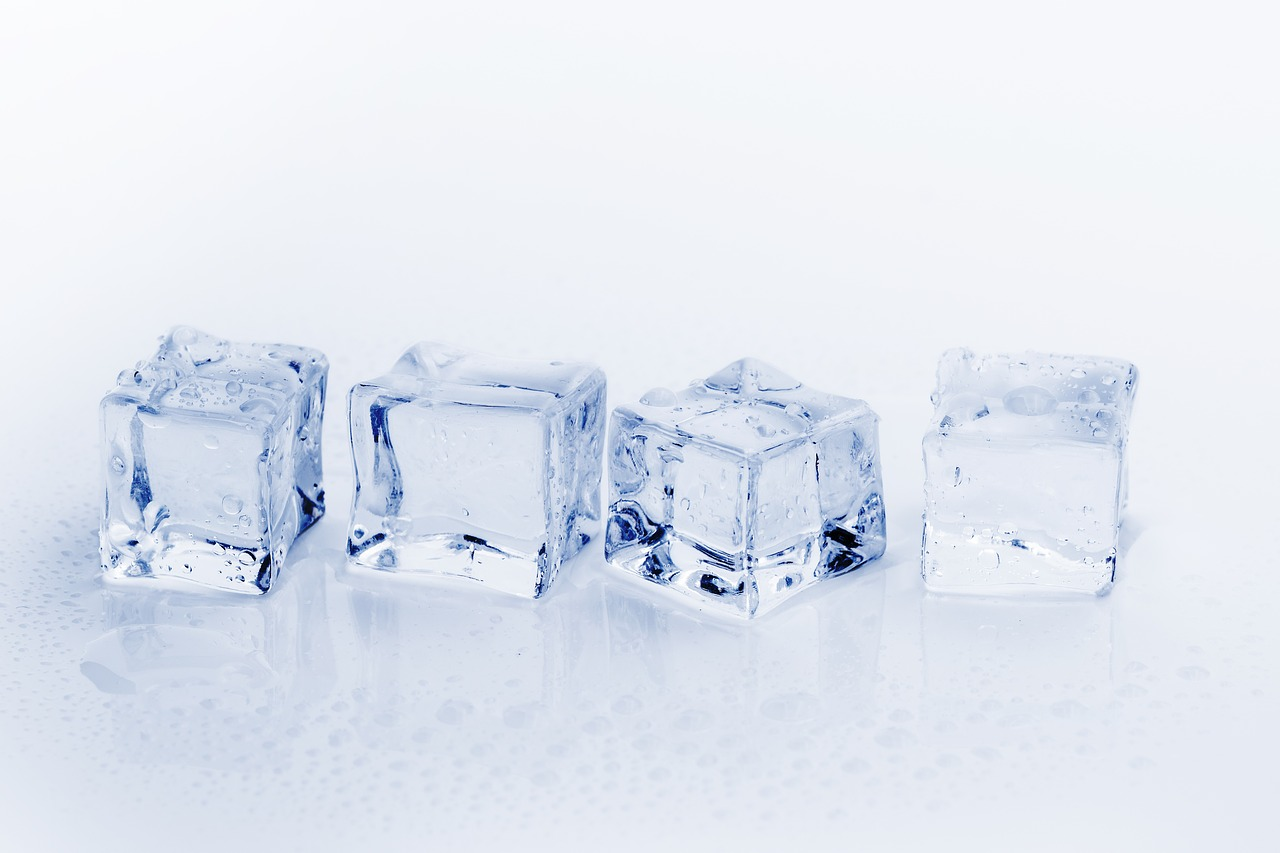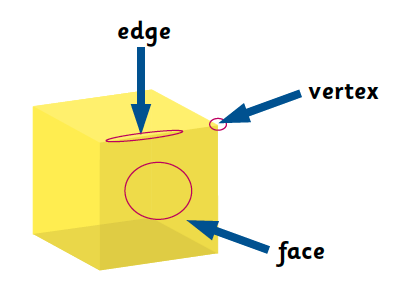# How to find volume of cube? Discover the parts of the cube

A cube is a solid of six congruent square faces, with all dimensions of the same length. The volume of a cube is measured in cubic units of measure. Think of dice or any other similarly shaped object of any size. The volume refers to the amount of space it occupies and if you know the size of one side of the cube, you can easily calculate its volume. So do not wait any longer and learn with this article How to find volume of cube.

Using the formula to calculate the volume of a cube you can remove it in a simple and easy way. You may also learn how to find volume of a rectangular prism?

## How to find volume of cube?

Measure the length of one of the sides or edges. It does not matter which side is measured since all the dimensions are equal, that is, it is a regular polyhedron with 6 equal faces. Normally, when carrying out mathematical exercises, they will always give you this information to be able to apply the cube volume formula.

The formula for calculating the volume of a cube is equal to the length of its edge raised to the cube: V = a³

In this way, you must multiply the side 3 times by itself.Suppose then that the edge of the cube whose volume we want to calculate measures 6 cm, so we must replace this value in the formula and perform the relevant mathematical operation:

V = 6³ = 6x6x6 = 216 cm³

So, we have already obtained the result of applying the formula to calculate the volume of the cube.

### Characteristics of the cube

• A cube has 6 faces.
• The number of vertices in a cube is 8.
• The cube has 12 edges in its composition.

### Find the volume of a cuboid

A cuboid has six sides or flat and rectangular faces. It is also distinguished in that its opposite faces are equal. The volume of a cuboid is obtained by multiplying the length by the width by the height. Using the following notation:

Volume, V
Long, L
Wide, A
Tall, h

We can express the formula to calculate the volume of a cuboid as:

V = L ⋅ A ⋅ h

Consider the following cuboid:

To calculate your volume we substitute the values ​​in the formula:

V = 5 [cm] ⋅ 2 [cm] ⋅ 3 [cm]

We perform the calculations:

V = 5 ⋅ 2 ⋅ 3 [cm ⋅ cm ⋅ cm]

Finally, we obtain that the volume of the cuboid is equal to 30 cubic centimeters.

V = 30 [cm³]

If the six faces are equal it is a cube.

### Parts of the cubeIn the area of ​​geometry, a face consists of each of the planes that are part of a dihedral or polyhedron angle, or also each of the polygons that make up or restrict a polyhedron. An example of this is that any of the squares that condition a regular hexahedron or cube is one side of it.

#### Base

The base refers to the lower side (which at the same time is horizontal) of a geometric figure of flat type (a triangle, trapezoid or parallelogram can be some examples). Its length is used to measure the area of ​​said figure.

#### Edge

It is known as edge to that line produced by the crossing between two planes. Edges are also defined as the segments of a line that point to the boundary of the sides of a flat figure. There is the possibility of linking the concept of edge to the notion of edge.

#### Vertex

The vertex covers an important notion in ​​geometry because this word is used to describe at all points where two segments that produce an angle intersect, as well as to the space where at least three planes are associated. The top of a pyramid or cone (consisting of three-dimensional geometric shapes) or the highest or lowest point of a curved line also the vertices.

#### Diagonal

The concept of diagonal is used to talk about that straight line that facilitates the union between two vertices that are close to a polygon or polyhedron. The diagonals are shown as straight lines that have a specific inclination.

#### Center

In the area of ​​geometry, the center is the point that is located in the middle of a geometric figure. It is a term whose meaning changes depending on the figure that is spoken of, as well as if there is an allusion to figures of three or two dimensions. The center of a line is the point that is in the middle of that segment.

Always remember to be careful with the units of measurement that you use in the calculations and the result should be expressed in cubic units. In this case, since the unit of length in which someone measured the edge were the centimeters, the result of the volume of the cube must be indicated in cubic centimeters.

#### Be the first to comment

This site uses Akismet to reduce spam. Learn how your comment data is processed.